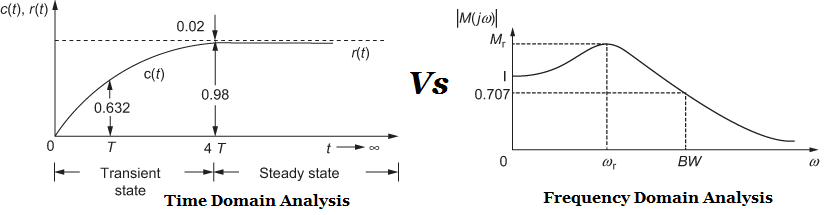# Difference between Time Domain Vs Frequency Domain Analysis## Time Domain Analysis Vs Frequency Domain Analysis

In this post, the major differences are shown between Time Domain Analysis Vs Frequency Domain Analysis. Before that, some short definitions of Time Domain Analysis & Frequency Domain Analysis are given.

## Time Response of the Control System

In control system Time Domain of a control system means, how output behaves with respect to time. So it can be defined as below:

Definition: The response given by the system which is function of the time, to the applied excitation is called time response of a control system.

Time response analysis is also known as time domain analysis & its’ output as a function of time.

## Frequency Response Analysis

Definition of Frequency Response Analysis: The steady state response of a system to a purely sinusoidal input is defined as frequency response of a system. In such method frequency of the input signal is to be varied over a certain range and the resulting response of system is to be studied. Such response is called frequency response.

Frequency response analysis is also known as frequency domain analysis & its output as a function of frequency.

## Comparison of time domain and frequency domain analysis is given:

1. Variable frequency, sinusoidal signal generators are readily available and precision measuring instruments are available for measurement of magnitude and phase angle. The time response for a step input is more difficult to measure with accuracy.
2. It is easier to obtain the transfer function of a system by a simple frequency domain test. Obtaining the transfer function from the step response is more tedious.
3. If the system has large time constants, it makes more time to reach a steady-state at each frequency of the sinusoidal input. Hence the time-domain method is preferred over the frequency-domain method in such systems.
4. In order to do a frequency response test on a system, the system has to be isolated and the sinusoidal signal has to be applied to the system. This may not be possible in systems that can not be interrupted. In such cases, a step signal or an impulse signal may be given to the system to find its transfer function. Hence for systems that cannot be interrupted, the time-domain method is more suitable.
5. The design of a controller is easily done in the frequency domain method than in the time-domain method. For a given set of performance measures in the frequency domain. the parameters of the open-loop transfer function can be adjusted easily using techniques to be discussed later in this chapter.
6. The effect of noise signals can be assessed easily in the frequency domain rather than the time domain.
7. The most important advantage of frequency domain analysis is the ability to obtain the relative stability of feedback control systems. The Routh Hurwitz criterion is essentially a time-domain method that determines the absolute stability of a system.